# Select a row of series or dataframe by given integer index

• Last Updated : 28 Jul, 2020

Dataframe.iloc[]  is used to select a row of series/dataframe by given integer index. Let’s Create a Data Frame:

Code:

## Python3

 `# import pandas library ` `import` `pandas as pd  ` ` `  `# Create the dataframe  ` `df ``=` `pd.DataFrame({``'ID'``: [``'114'``, ``'345'``, ` `                         ``'157788'``, ``'5626'``],  ` `                ``'Product'``: [``'shirt'``, ``'trousers'``, ` `                           ``'tie'``, ``'belt'``],  ` `                ``'Price'``: [``1200``, ``1500``, ` `                         ``600``, ``352``],  ` `                ``'Color'``: [``'White'``,``'Black'``,  ` `                         ``'Red'``, ``'Brown'``],  ` `                ``'Discount'``: [``10``, ``10``, ` `                            ``10``, ``10``]})  ` ` `  `# Show the dataframe ` `df `

Output: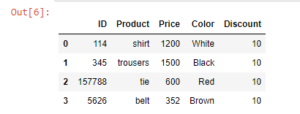Now, Selecting a row of series/dataframe by a given integer index:
Example 1: Selecting the first row only.

## Python3

 `# select first row  ` `# from the dataframe ` `df.iloc[``0``]`

Output: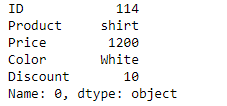Example 2: Selecting  0,1,2  rows.

## Python3

 `# select 0, 1, 2 rows ` `#from the dataframe ` `df.iloc[``0` `: ``3``]`

Output: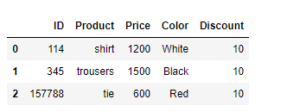Example 3: Selecting rows from 0 to 2 and columns 0 to 1.

## Python3

 `# selecting rows from 0 to ` `# 2 and columns 0 to 1 ` `df.iloc[``0` `: ``3``, ``0` `: ``2``]`

Output: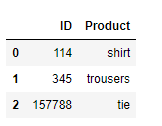Example 4: Selecting all rows and columns from 0 to 3.

## Python3

 `# selecting all rows and  ` `# columns from 0 to 3 ` `df.iloc[ : , ``0` `: ``4``] `

Output: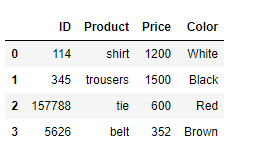Example 5: Selecting all rows and 2nd column.

## Python3

 `# selecting all rows and  ` `# 3rd column ` `df.iloc[ : , ``2``]`

Output: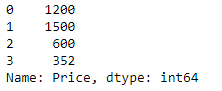My Personal Notes arrow_drop_up
Recommended Articles
Page :